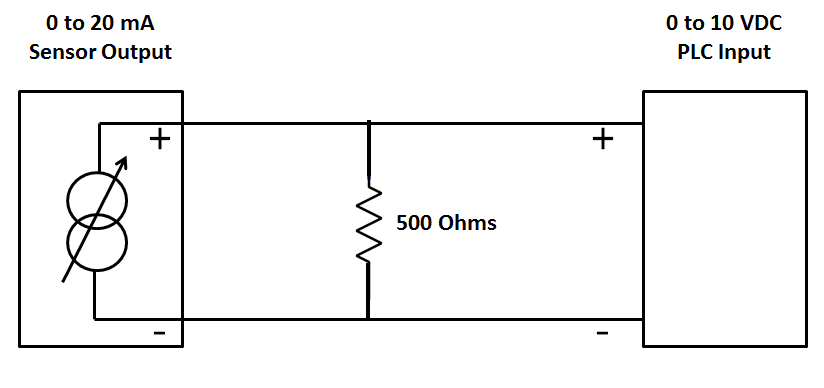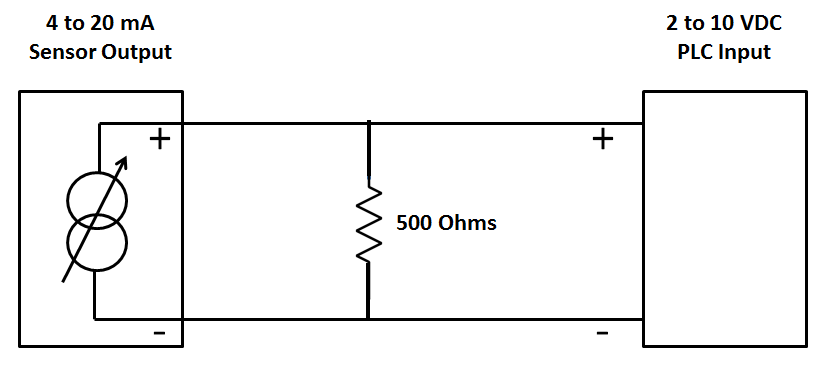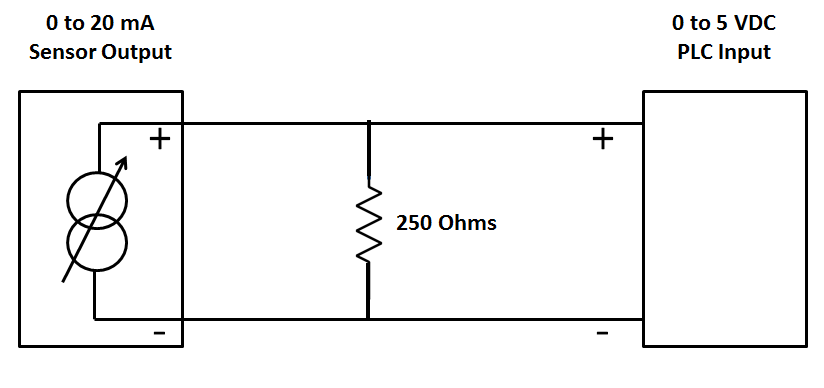### Convert 0-20 mA to 0-10 V Using Resistor

It is extremely simple to measure 0-20ma signal with a device that will measure only Voltage inputs. If Voltage input module available will accept a 0-10Vdc signal, but may not accept a 0-20ma signal directly.

Basically, Ohms law is used to calculate a resistor value in order to convert the 0-20ma signal to a voltage.

Current to Voltage Conversion Circuit Diagram

#### Example (0-20 mA to 0-10 VDC)

Ohms law states: R = V/I where V is the Voltage, I is the current and R is the resistance

R = 10V/0.020A = 500 Ohms0-20 mA to 0-10 VDC Conversion Circuit Diagram

V = I*R = 0*500        = 0V

V = I*R = 0.020*500 = 10V

#### Example (4-20 mA to 2-10 VDC)

Ohms law states: R = V/I where V is the Voltage, I is the current and R is the resistance

R = 10V/0.020A = 500 Ohms4-20 mA to 2-10 VDC Conversion Circuit Diagram

V = I*R = 0.004*500 = 2V

V = I*R = 0.020*500 = 10V

#### Example (0-20 mA to 0-5 VDC)

Ohms law states: R = V/I where V is the Voltage, I is the current and R is the resistance

R = 5V/0.020A = 250 Ohms0-20 mA to 0-5 VDC Conversion Circuit Diagram

V = I*R = 0*250        = 0V

V = I*R = 0.020*250 = 5V

#### Note:-

To avoid damage you must ensure that the external current source has short-circuit protection in all conductor cases.

The external resistor is a source of error because of its dependency on temperature and its inaccuracy.
In order to obtain measuring results that are as precise as possible it is recommended to use resistors with tolerances that are as small as possible.

1.2.3.